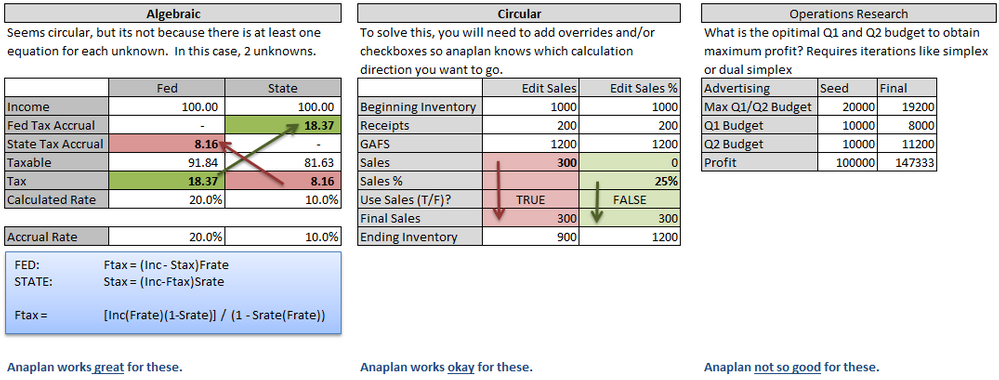# Circular references Cash flow planningHello!

The model that I’m building for the client includes circular reference. I need to calculate the amount of the loans  and net debt that depend on the Free cash flow sum for the period. Free cash flow at the same time includes interests paid, that is calculated for the net debt. When i'm changing any parametrs that effects FCF net Debt and interests is changing according to this for the current and future years. In Excel it’s calculates with Enable iterative calculation option on. Does anyone could help me with solutions and best practices for this case in Anaplan? I suppose it’s a common case with financial planning models.

Tagged:

•Although I'm not a fan of this solution, here's what I've done in the past to get around circular references:  In one case, it was just a few numbers, so we had a little dashboard where we'd copy/paste the base numbers into other line items to make them (effectively) fixed... and then use the copy/pasted numbers in subsequent calculations.  In another model, we had a button to do (effectively) the same thing.  Either way, the FP&A folks would update this as needed.

•+1 Kuddo for this question.  It's one that I spent a lot of time with when I first started building planning models for merchandis planning (retail industry).

There's no easy way to solve your problem using Anaplan unless there is an underlying equation that can be defined.  I've not had much luck with trying to find optimal solutions.

I couldn't tell precisely what you were trying to accomplish but it looked like you are discounting loans and FCF across time which means there's an underlying equation.  Below is a job aid I created for our company that helps explain the different kinds of circular references:

• Algebraic - Same number of equations as unknowns. Anaplan can handle great.
• Circular - Multiple editable line items for same equation.  Anaplan is okay, but you will need to add a lot of line items.
• Operations Research (optimal solves) - Anaplan not so great for these.

Here's a handy diagram to help explain these three.•If the calculation is truly circular such that you cannot compute one value without referencing a dependent value in the same period then one approach could be to use a staging data import process to break the dependencies.

So in your case you would do something like this:

• Calculate net debt to provide an interim net debt value.
• Run an import process to store this interim net debt value on a line item
• Calculate amount of loans based on the interim net debt (the values that you populated via the import).

The issue you will however run into with this approach is that you will need to iterate the import and calculation to get to the final result. This could be done by duplicating the import action and popping a couple of these into a process.

I can't follow the exact flow of your logic so apologies if the order is not quite right, however the principle should stand.

•This is a great question and is probably prevalent in 3 statement financial models or banking models. And excel does solve using iterative calcuations. @darsid -- please let me know if you have figured an answer for this.